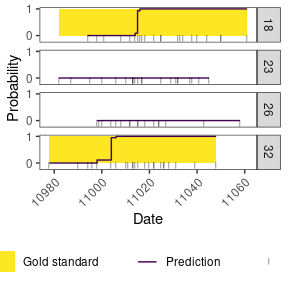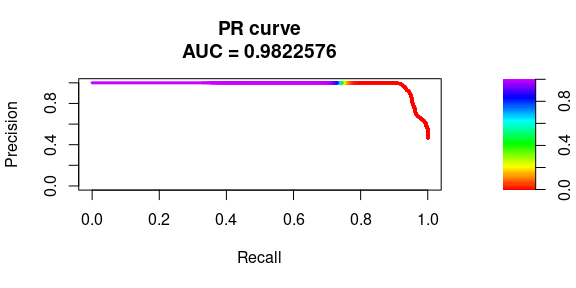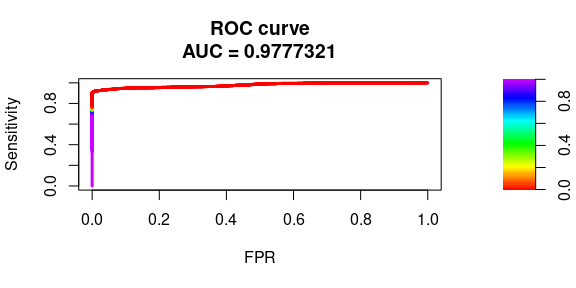Tuto PheVis

Introduction

Welcome to PheVis package, an unsupervised R package for phenotyping at visit resolution. I will briefly explain how it works, for more details about the underlying method. The detailed method is available on MedRxiv (doi: https://doi.org/10.1101/2020.06.15.20131458)

Toy dataset

First we need a toy dataset, luckily, there is one provided inside the package. Here are the first rows and columns of the dataset.

library(PheVis)
library(dplyr)
library(knitr)
library(ggplot2)

data("data_phevis")

subject time PR_state mainICD mainCUI var1 var2
1 2000-01-11 1 1 12 10 0
1 2000-02-06 1 1 2 9 1
1 2000-02-08 1 0 6 8 1
1 2000-02-23 1 0 6 10 1
1 2000-02-25 1 1 11 7 1
1 2000-02-27 1 2 10 11 2

We are going to split it in train and test set, add an ENCOUNTER_NUM column (one identifier by visit) and convert date to numeric format.

df <- data_phevis %>%
mutate(ENCOUNTER_NUM = row_number(),
time = round(as.numeric(time)))

set.seed(1)

trainsize <- 0.8*length(unique(df$subject)) trainid <- sample(x = unique(df$subject), size = trainsize)
testid <- unique(df$subject)[!unique(df$subject) %in% trainid]

df_train <- as.data.frame(df[df$subject %in% trainid,]) df_test <- as.data.frame(df[df$subject %in% testid,])

Train PheVis

To train PheVis we first should set the parameters that are used by the algorithm:

• The variables used for the prediction var_vec
• The main surrogates highly predictive of the phenotype of interest: main_icd and main_cui
• The gold-standard variable used only to evaluate the performance of PheVis (i.e not needed for model training): GS
• The half_life of the cumulative decay, you can use the disease duration. Here we consider a chronic disease: half_life
var_vec <- c(paste0("var",1:10), "mainCUI", "mainICD")
main_icd <- "mainICD"
main_cui <- "mainCUI"
GS <- "PR_state"
half_life <- Inf

Now we train the model, it might take a while.

train_model <- PheVis::train_phevis(half_life = half_life,
df = df_train,
START_DATE = "time",
PATIENT_NUM = "subject",
ENCOUNTER_NUM = "ENCOUNTER_NUM",
var_vec = var_vec,
main_icd = main_icd,
main_cui = main_cui)
#> -- Arguments passed check -> PheVis begins --
#> Joining, by = c("id", "id_encounter", "var", "start_date")
#> Joining, by = c("PATIENT_NUM", "ENCOUNTER_NUM")
#> Joining, by = c("START_DATE", "PATIENT_NUM", "ENCOUNTER_NUM")

Test PheVis

Now that we have trained the model, we are going to use it to get probabilities prediction for the test set. It also takes a while as the cumulated variables must be computed for the test dataset too.

test_model <- PheVis::test_phevis(train_param = train_model$train_param, df_test = df_test, START_DATE = "time", PATIENT_NUM = "subject", ENCOUNTER_NUM = "ENCOUNTER_NUM", surparam = train_model$surparam,
model = train_model$model) #> -- Arguments passed check -> PheVis begins -- #> Joining, by = c("id", "id_encounter", "var", "start_date") #> Joining, by = c("PATIENT_NUM", "ENCOUNTER_NUM") #> Joining, by = c("START_DATE", "PATIENT_NUM", "ENCOUNTER_NUM") Results train_model We can access the different components of the returned objects. train_model is a list containing multiples objects. We have surparam, the parameters computed inside PheVis to compute the surrogate (mean and sd of main surrogates). train_model$surparam
#> $mean_icd #>  0.5073889 #> #>$sd_icd
#>  0.8720246
#>
#> $mean_nlp #>  4.532568 #> #>$sd_nlp
#>  4.554872
#>
#> $min_all #>  -1.576955 model which contains the fixed effect of the model and the type of model trained (usually glmer for mixed effect logistic regression but might be glm for logistic regression if mixed model fails to converge). train_model$model
#> $fixedEffect #> (Intercept) mainCUI mainICD var1 var4 #> -31.829321569 -0.041409214 -0.017401136 0.037806258 -0.015078208 #> var9 var10 var1_cum var4_cum var9_cum #> 0.082143743 -0.004552051 0.083685825 0.052506162 0.050956432 #> var10_cum mainCUI_cum mainICD_cum last_vis last_5vis #> 0.097538016 0.067701129 0.312671123 -0.102492184 0.303248452 #> cum cum_month cum_year #> 0.190078948 0.427306810 0.131637633 #> #>$model
#>  "glmer"

df_train_result the data.frame with the surrogates (qualitative and quantitative), the output probability and the visit id.

head(train_model$df_train_result) #> ENCOUNTER_NUM PRED SUR_QUANTI SUR_QUALI #> 1 1 1.593245e-11 3.781298 3 #> 2 2 1.777237e-09 5.367145 3 #> 3 3 1.072112e-07 6.684416 3 #> 4 4 1.422842e-06 8.001687 3 #> 5 5 2.292558e-04 11.563440 3 #> 6 6 5.327204e-01 16.052404 3 train_param corresponds to the hyperparameters of PheVis chosen by the user. train_model$train_param
#> $half_life #>  Inf #> #>$omega
#>  2
#>
#> $var_vec #>  "var1" "var2" "var3" "var4" "var5" "var6" "var7" #>  "var8" "var9" "var10" "mainCUI" "mainICD" #> #>$main_icd
#>  "mainICD"
#>
#> $main_cui #>  "mainCUI" #> #>$GS
#> NULL

df_x_train is the final data.frame to predict the probability with the cumulated features.

head(train_model$df_x_train[,c(1:2, 14:15, 29:30)]) #> var1 var2 var1_cum var2_cum cum_month cum_year #> 1 10 0 10 0 3.781298 3.781298 #> 2 9 1 19 1 5.367145 5.367145 #> 3 8 1 27 2 6.684416 6.684416 #> 4 10 1 37 3 4.220388 8.001687 #> 5 7 1 44 4 7.782141 11.563440 #> 6 11 2 55 6 12.271106 16.052404 test_model test_model is also a list containing two data.frame. We have df_result with the predictions of the model. head(test_model$df_result)
#>   PATIENT_NUM ENCOUNTER_NUM START_DATE SUR_QUANTI SUR_QUALI   PREDICTION
#> 1          18           347      10994   3.512722         3 2.592247e-12
#> 2          18           348      10998   6.854930         3 1.905790e-09
#> 3          18           349      11001   8.391746         3 3.516198e-07
#> 4          18           350      11008   9.928562         3 1.445644e-05
#> 5          18           351      11012  11.245832         3 4.459433e-04
#> 6          18           352      11014  14.539009         3 8.700906e-02

And df_pred the data.frame with the model predictions.

head(test_model$df_pred[,c(1:2, 14:15, 29:30)]) #> var1 var2 PATIENT_NUM ENCOUNTER_NUM last_5vis cum #> 1 6 3 18 347 0.000000 3.512722 #> 2 4 2 18 348 3.512722 6.854930 #> 3 7 2 18 349 6.854930 8.391746 #> 4 4 1 18 350 8.391746 9.928562 #> 5 10 0 18 351 9.928562 11.245832 #> 6 3 2 18 352 11.245832 14.539009 plot predictions We can display a graph with the prediction and the gold-standard with the function ggindividual_plot. df_plot <- test_model$df_result %>%
left_join(df_test) %>%
filter(PATIENT_NUM %in% c(18, 23, 26, 32))
#> Joining, by = "ENCOUNTER_NUM"

PheVis::ggindividual_plot(subject = df_plot$PATIENT_NUM, time = df_plot$START_DATE,
gold_standard = df_plot$PR_state, prediction = df_plot$PREDICTION)Performances

Now we can see the performance of the model using ROC cure and Precision Recall (PR) curve

pr_curve <-PRROC::pr.curve(scores.class0 = test_model$df_result$PREDICTION,
weights.class0 = df_test$PR_state, curve = TRUE) plot(pr_curve)roc_curve <- PRROC::roc.curve(scores.class0 = test_model$df_result$PREDICTION, weights.class0 = df_test$PR_state,
curve = TRUE)
plot(roc_curve)